# mne.compute_covariance#

mne.compute_covariance(epochs, keep_sample_mean=True, tmin=None, tmax=None, projs=None, method='empirical', method_params=None, cv=3, scalings=None, n_jobs=None, return_estimators=False, on_mismatch='raise', rank=None, verbose=None)[source]#

Estimate noise covariance matrix from epochs.

The noise covariance is typically estimated on pre-stimulus periods when the stimulus onset is defined from events.

If the covariance is computed for multiple event types (events with different IDs), the following two options can be used and combined:

1. either an Epochs object for each event type is created and a list of Epochs is passed to this function.

2. an Epochs object is created for multiple events and passed to this function.

Note

To estimate the noise covariance from non-epoched raw data, such as an empty-room recording, use `mne.compute_raw_covariance()` instead.

Parameters:
epochsinstance of `Epochs`, or `list` of `Epochs`

The epochs.

keep_sample_mean`bool` (default `True`)

If False, the average response over epochs is computed for each event type and subtracted during the covariance computation. This is useful if the evoked response from a previous stimulus extends into the baseline period of the next. Note. This option is only implemented for method=’empirical’.

tmin

Start time for baseline. If None start at first sample.

tmax

End time for baseline. If None end at last sample.

projs

List of projectors to use in covariance calculation, or None to indicate that the projectors from the epochs should be inherited. If None, then projectors from all epochs must match.

method`str` | `list` | `None` (default ‘empirical’)

The method used for covariance estimation. If ‘empirical’ (default), the sample covariance will be computed. A list can be passed to perform estimates using multiple methods. If ‘auto’ or a list of methods, the best estimator will be determined based on log-likelihood and cross-validation on unseen data as described in . Valid methods are ‘empirical’, ‘diagonal_fixed’, ‘shrunk’, ‘oas’, ‘ledoit_wolf’, ‘factor_analysis’, ‘shrinkage’, and ‘pca’ (see Notes). If `'auto'`, it expands to:

```['shrunk', 'diagonal_fixed', 'empirical', 'factor_analysis']
```

`'factor_analysis'` is removed when `rank` is not ‘full’. The `'auto'` mode is not recommended if there are many segments of data, since computation can take a long time.

New in version 0.9.0.

method_params

Additional parameters to the estimation procedure. Only considered if method is not None. Keys must correspond to the value(s) of `method`. If None (default), expands to the following (with the addition of ```{'store_precision': False, 'assume_centered': True} for all methods except ``'factor_analysis'``` and `'pca'`):

```{'diagonal_fixed': {'grad': 0.1, 'mag': 0.1, 'eeg': 0.1, ...},
'shrinkage': {'shrikage': 0.1},
'shrunk': {'shrinkage': np.logspace(-4, 0, 30)},
'pca': {'iter_n_components': None},
'factor_analysis': {'iter_n_components': None}}
```
cv`int` | `sklearn.model_selection` object (default 3)

The cross validation method. Defaults to 3, which will internally trigger by default `sklearn.model_selection.KFold` with 3 splits.

scalings

Defaults to `dict(mag=1e15, grad=1e13, eeg=1e6)`. These defaults will scale data to roughly the same order of magnitude.

n_jobs

The number of jobs to run in parallel. If `-1`, it is set to the number of CPU cores. Requires the `joblib` package. `None` (default) is a marker for ‘unset’ that will be interpreted as `n_jobs=1` (sequential execution) unless the call is performed under a `joblib.parallel_backend()` context manager that sets another value for `n_jobs`.

return_estimators`bool` (default `False`)

Whether to return all estimators or the best. Only considered if method equals ‘auto’ or is a list of str. Defaults to False.

on_mismatch`str`

What to do when the MEG<->Head transformations do not match between epochs. If “raise” (default) an error is raised, if “warn” then a warning is emitted, if “ignore” then nothing is printed. Having mismatched transforms can in some cases lead to unexpected or unstable results in covariance calculation, e.g. when data have been processed with Maxwell filtering but not transformed to the same head position.

rank`None` | ‘info’ | ‘full’ | `dict`

This controls the rank computation that can be read from the measurement info or estimated from the data. When a noise covariance is used for whitening, this should reflect the rank of that covariance, otherwise amplification of noise components can occur in whitening (e.g., often during source localization).

`None`

The rank will be estimated from the data after proper scaling of different channel types.

`'info'`

The rank is inferred from `info`. If data have been processed with Maxwell filtering, the Maxwell filtering header is used. Otherwise, the channel counts themselves are used. In both cases, the number of projectors is subtracted from the (effective) number of channels in the data. For example, if Maxwell filtering reduces the rank to 68, with two projectors the returned value will be 66.

`'full'`

The rank is assumed to be full, i.e. equal to the number of good channels. If a `Covariance` is passed, this can make sense if it has been (possibly improperly) regularized without taking into account the true data rank.

`dict`

Calculate the rank only for a subset of channel types, and explicitly specify the rank for the remaining channel types. This can be extremely useful if you already know the rank of (part of) your data, for instance in case you have calculated it earlier.

This parameter must be a dictionary whose keys correspond to channel types in the data (e.g. `'meg'`, `'mag'`, `'grad'`, `'eeg'`), and whose values are integers representing the respective ranks. For example, `{'mag': 90, 'eeg': 45}` will assume a rank of `90` and `45` for magnetometer data and EEG data, respectively.

The ranks for all channel types present in the data, but not specified in the dictionary will be estimated empirically. That is, if you passed a dataset containing magnetometer, gradiometer, and EEG data together with the dictionary from the previous example, only the gradiometer rank would be determined, while the specified magnetometer and EEG ranks would be taken for granted.

The default is `None`.

New in version 0.17.

New in version 0.18: Support for ‘info’ mode.

verbose

Control verbosity of the logging output. If `None`, use the default verbosity level. See the logging documentation and `mne.verbose()` for details. Should only be passed as a keyword argument.

Returns:
covinstance of `Covariance` | `list`

The computed covariance. If method equals ‘auto’ or is a list of str and return_estimators equals True, a list of covariance estimators is returned (sorted by log-likelihood, from high to low, i.e. from best to worst).

`compute_raw_covariance`

Estimate noise covariance from raw data, such as empty-room recordings.

Notes

Baseline correction or sufficient high-passing should be used when creating the `Epochs` to ensure that the data are zero mean, otherwise the computed covariance matrix will be inaccurate.

Valid `method` strings are:

• `'empirical'`

The empirical or sample covariance (default)

• `'diagonal_fixed'`

A diagonal regularization based on channel types as in `mne.cov.regularize()`.

• `'shrinkage'`

Fixed shrinkage.

New in version 0.16.

• `'ledoit_wolf'`

The Ledoit-Wolf estimator, which uses an empirical formula for the optimal shrinkage value .

• `'oas'`

The OAS estimator , which uses a different empricial formula for the optimal shrinkage value.

New in version 0.16.

• `'shrunk'`

Like ‘ledoit_wolf’, but with cross-validation for optimal alpha.

• `'pca'`

Probabilistic PCA with low rank .

• `'factor_analysis'`

Factor analysis with low rank .

`'ledoit_wolf'` and `'pca'` are similar to `'shrunk'` and `'factor_analysis'`, respectively, except that they use cross validation (which is useful when samples are correlated, which is often the case for M/EEG data). The former two are not included in the `'auto'` mode to avoid redundancy.

For multiple event types, it is also possible to create a single `Epochs` object with events obtained using `mne.merge_events()`. However, the resulting covariance matrix will only be correct if `keep_sample_mean is True`.

The covariance can be unstable if the number of samples is small. In that case it is common to regularize the covariance estimate. The `method` parameter allows to regularize the covariance in an automated way. It also allows to select between different alternative estimation algorithms which themselves achieve regularization. Details are described in .

References

## Examples using `mne.compute_covariance`#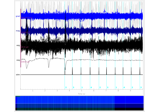Plotting whitened data

Plotting whitened data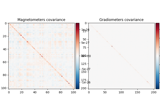Computing a covariance matrix

Computing a covariance matrix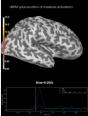Source localization with MNE, dSPM, sLORETA, and eLORETA

Source localization with MNE, dSPM, sLORETA, and eLORETA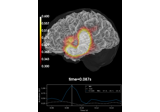Source reconstruction using an LCMV beamformer

Source reconstruction using an LCMV beamformer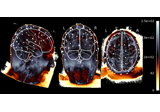EEG source localization given electrode locations on an MRI

EEG source localization given electrode locations on an MRI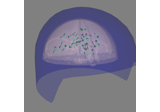Brainstorm Elekta phantom dataset tutorial

Brainstorm Elekta phantom dataset tutorial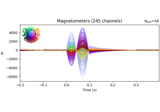4D Neuroimaging/BTi phantom dataset tutorial

4D Neuroimaging/BTi phantom dataset tutorial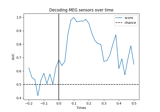Decoding (MVPA)

Decoding (MVPA)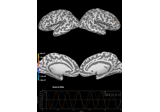Corrupt known signal with point spread

Corrupt known signal with point spread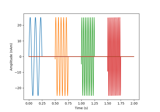Generate simulated raw data

Generate simulated raw data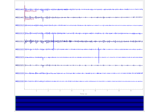Plot sensor denoising using oversampled temporal projection

Plot sensor denoising using oversampled temporal projection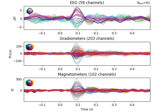Whitening evoked data with a noise covariance

Whitening evoked data with a noise covariance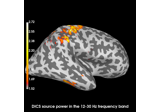Compute evoked ERS source power using DICS, LCMV beamformer, and dSPM

Compute evoked ERS source power using DICS, LCMV beamformer, and dSPM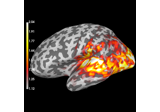Compute source power estimate by projecting the covariance with MNE

Compute source power estimate by projecting the covariance with MNE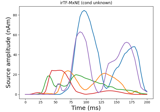Compute iterative reweighted TF-MxNE with multiscale time-frequency dictionary

Compute iterative reweighted TF-MxNE with multiscale time-frequency dictionary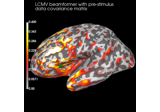Compute cross-talk functions for LCMV beamformers

Compute cross-talk functions for LCMV beamformers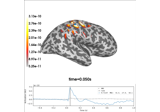Optically pumped magnetometer (OPM) data

Optically pumped magnetometer (OPM) dataFrom raw data to dSPM on SPM Faces dataset

From raw data to dSPM on SPM Faces dataset